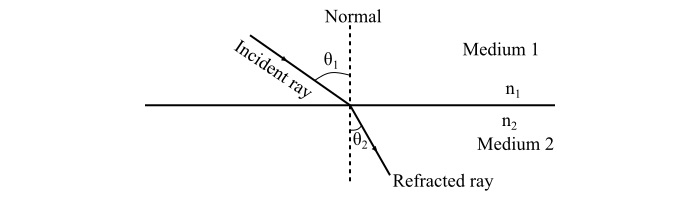# What are the Basic Principles of Light Control?

Light is the natural agent that simulates sight and makes the things visible. Light is also known as visible electromagnetic radiation as it is capable of causing a visual sensation and its wavelength varies from 380 nanometers to 780 nanometers.

## Characteristics of Light

The main characteristics of light are given as follows −

• Light is nothing but a form of energy produced by a luminous object.

• It is an electromagnetic radiation, thus it can travel through vacuum.

• In an optically homogeneous medium, light travels in a straight line.

• When light falls on a polished surface such as mirror or metal surface, it bounces back. This bouncing back of light is known as reflection of light.

• Light can penetrate through a transparent object but it cannot pass through an opaque object.

• When light goes from one transparent medium to another, there is a change occurs in the velocity of the light, this phenomenon is known as refraction of light.

## Basic Principles of Light Control

Depending upon the characteristics of light, discussed above, there are following four method of light control viz −

• Reflection

• Refraction

• Diffusion

• Absorption

## Reflection

The phenomenon in which light falling on a surface is sent back to the same medium is known as reflection. The ratio of reflected light energy to the incident light energy is known as reflection factor.

The law of reflection states that “the angle of incidence is equal to the angle of reflection.”

By using the law of reflection, the amount of light can be easily controlled.

There are two basic types of reflection, viz −

• Regular or Mirror or Specular Reflection

• Irregular or Diffuse Reflection

Regular Reflection

In case of regular reflection, a beam of light is reflected but not scattered. The regular reflection takes place when a beam of light is incident on a polished surface such as a mirror or highly polished metal surface, etc. In this case, the reflected light beam moves only in a fixed direction.Irregular or Diffuse Reflection

The diffuse reflection of light takes place when a ray of light falls on a rough surface like a wood or a wall, etc. In the diffused reflection, the reflected light is scattered in all the directions and the viewer sees the illuminated surface not the source of light.

## Refraction

When a ray of light is passed through a boundary between two transparent mediums having different densities, its velocity and direction be changed, this phenomenon is known as refraction.The law of refraction, known as Snell's law, states that

$$\mathrm{\mathit{n_{\mathrm{1}}}\:\mathrm{sin}\:\theta _{\mathrm{1}}\:=\:\mathit{n_{\mathrm{2}}}\:\mathrm{sin}\:\theta _{\mathrm{2}}}$$

Where,

• '$\mathit{n_{\mathrm{1}}}$' is the refractive index of medium 1.

• '$\mathit{n_{\mathrm{2}}}$' is the refractive index of medium 2.

• '$\theta _{\mathrm{1}}$' is the angle of light in medium 1.

• '$\theta _{\mathrm{2}}$' is the angle of light in medium 2.

By changing the angle of incidence or refraction, the light falling on a surface can be controlled.

## Diffusion

When light is incident on a rough surface and it is reflected or transmitted in many directions at once, this phenomenon is known as diffusion. The diffusion of light is also called scattering.

To control the light, i.e., in order to prevent the glare from a light source, a diffusion glass screen can be introduced between the viewer and the source of light.

The diffusion glass employed for light control are of two types viz −

• Opal Glass - Opal glass is the glass made of a material having refractive index different from that of an ordinary glass. The milk glass is an example of opal glass.

• Frosted Glass - Frosted glass is produced by coating an ordinary glass with a crystalline substance.

## Absorption

The phenomenon of taking up and storing of light energy, without it being reflected or transmitted is known as absorption. The absorption of light takes place when the beam of light falls on a surface and the energy from the light ray is transferred to the material of the surface.

During the phenomenon of absorption of light, the light energy may change from one form to another. The surface that absorbs light prevents the reflection or diffusion of light falling on the surface. Therefore, by employing the absorption of light, the glare of light on a surface can be controlled.

Updated on: 04-Apr-2022

2K+ Views